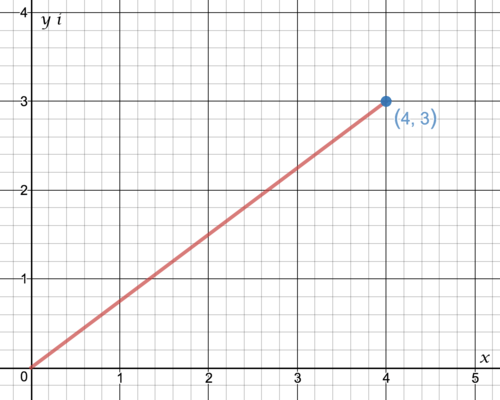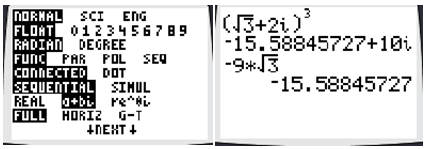# 11.2 Arithmetic with Complex Numbers

$$\newcommand{\vecs}{\overset { \rightharpoonup} {\mathbf{#1}} }$$ $$\newcommand{\vecd}{\overset{-\!-\!\rightharpoonup}{\vphantom{a}\smash {#1}}}$$$$\newcommand{\id}{\mathrm{id}}$$ $$\newcommand{\Span}{\mathrm{span}}$$ $$\newcommand{\kernel}{\mathrm{null}\,}$$ $$\newcommand{\range}{\mathrm{range}\,}$$ $$\newcommand{\RealPart}{\mathrm{Re}}$$ $$\newcommand{\ImaginaryPart}{\mathrm{Im}}$$ $$\newcommand{\Argument}{\mathrm{Arg}}$$ $$\newcommand{\norm}{\| #1 \|}$$ $$\newcommand{\inner}{\langle #1, #2 \rangle}$$ $$\newcommand{\Span}{\mathrm{span}}$$ $$\newcommand{\id}{\mathrm{id}}$$ $$\newcommand{\Span}{\mathrm{span}}$$ $$\newcommand{\kernel}{\mathrm{null}\,}$$ $$\newcommand{\range}{\mathrm{range}\,}$$ $$\newcommand{\RealPart}{\mathrm{Re}}$$ $$\newcommand{\ImaginaryPart}{\mathrm{Im}}$$ $$\newcommand{\Argument}{\mathrm{Arg}}$$ $$\newcommand{\norm}{\| #1 \|}$$ $$\newcommand{\inner}{\langle #1, #2 \rangle}$$ $$\newcommand{\Span}{\mathrm{span}}$$$$\newcommand{\AA}{\unicode[.8,0]{x212B}}$$

The idea of a complex number can be hard to comprehend, especially when you start thinking about absolute value. In the past you may have thought of the absolute value of a number as just the number itself or its positive version. How should you think about the absolute value of a complex number?

## Arithmetic Operations with Complex Numbers

Complex numbers follow all the same rules as real numbers for the operations of adding, subtracting, multiplying and dividing. There are a few important ideas to remember when working with complex numbers:

1. When simplifying, you must remember to combine imaginary parts with imaginary parts and real parts with real parts. For example, $$4+5 i+2-3 i=6+2 i$$

2. If you end up with a complex number in the denominator of a fraction, eliminate it by multiplying both the numerator and denominator by the complex conjugate of the denominator.

3. The powers of $$i$$ are:

• $$i=\sqrt{-1}$$
• $$i^{2}=-1$$
• $$i^{3}=-\sqrt{-1}=-i$$
• $$i^{4}=1$$
• $$i^{5}=i$$
• $$\ldots$$ and the pattern repeats

Consider this complex expression:

$$(2+3 i)(1-5 i)-3 i+8$$

First, multiply the two binomials and then combine the imaginary parts with imaginary parts and real parts with real parts.

$$=2-10 i+3 i-15 i^{2}-3 i+8$$

$$=10-10 i+15$$

$$=25-10 i$$

Note that a power higher than 1 of $$i$$ can be simplified using the pattern above.

The complex plane is set up in the same way as the regular $$x, y$$ plane, except that real numbers are counted horizontally and complex numbers are counted vertically. The following is the number $$4+3 i$$ plotted in the complex number plane. Notice how the point is four units over and three units up.The absolute value of a complex number like $$|4+3 i|$$ is defined as the distance from the complex number to the origin. You can use the Pythagorean Theorem to get the absolute value. In this case, $$|4+3 i|=\sqrt{4^{2}+3^{2}}=\sqrt{25}=5$$.

## Examples

##### Example 1

Earlier, you were asked how to think about the absolute value of a complex number. A good way to think about the absolute value for all numbers is to define it as the distance from a number to zero. In the case of complex numbers where an individual number is actually a coordinate on a plane, zero is the origin.

##### Example 2

Compute the following power by hand and use your calculator to support your work.

$$(\sqrt{3}+2 i)^{3}$$

$$(\sqrt{3}+2 i) \cdot(\sqrt{3}+2 i) \cdot(\sqrt{3}+2 i)$$

$$=(3+4 i \sqrt{3}-4)(\sqrt{3}+2 i)$$

$$=(-1+4 i \sqrt{3})(\sqrt{3}+2 i)$$

$$=-\sqrt{3}-2 i+12 i-8 \sqrt{3}$$

$$=-9 \sqrt{3}+10 i$$

A TI-84 can be switched to imaginary mode and then compute exactly what you just did. Note that the calculator will give a decimal approximation for $$-9 \sqrt{3}$$.##### Example 3

Simplify the following complex expression.

$$\frac{7-9 i}{4-3 i}+\frac{3-5 i}{2 i}$$

To add fractions you need to find a common denominator.

$$\frac{(7-9 i) \cdot 2 i}{(4-3 i) \cdot 2 i}+\frac{(3-5 i) \cdot(4-3 i)}{2 i \cdot(4-3 i)}$$

$$=\frac{14 i+18}{8 i+6}+\frac{12-20 i-9 i-15}{8 i+6}$$

$$=\frac{15-15 i}{8 i+6}$$

Lastly, eliminate the imaginary component from the denominator by using the conjugate.

$$=\frac{(15-15 i) \cdot(8 i-6)}{(8 i+6) \cdot(8 i-6)}$$

$$=\frac{120 i-90+120+90 i}{100}$$

$$=\frac{30 i+30}{100}$$

$$=\frac{3 i+3}{10}$$

##### Example 4

Simplify the following complex number.

$$i^{2013}$$

When simplifying complex numbers, $$i$$ should not have a power greater than 1 . The powers of $$i$$ repeat in a four part cycle:

$$i^{5}=i=\sqrt{-1}$$

$$i^{6}=i^{2}=-1$$

$$i^{7}=i^{3}=-\sqrt{-1}=-i$$

$$i^{8}=i^{4}=1$$

Therefore, you just need to determine where 2013 is in the cycle. To do this, determine the remainder when you divide 2013 by 4 . The remainder is 1 so $$i^{2013}=i$$.

##### Example 5

Plot the following complex number on the complex coordinate plane and determine its absolute value.

$$-12+5 i$$The sides of the right triangle are 5 and $$12,$$ which you should recognize as a Pythagorean

triple with a hypotenuse of 13. $$|-12+5 i|=13$$.

##### Review

Simplify the following complex numbers.

1. $$i^{252}$$

2. $$i^{312}$$

3. $$i^{411}$$

4. $$i^{2345}$$

For each of the following, plot the complex number on the complex coordinate plane and

determine its absolute value.

5. $$6-8 i$$

6. $$2+i$$

7. $$4-2 i$$

8. $$-5 i+1$$

Let $$c=2+7 i$$ and $$d=3-5 i$$

9. What is $$c+d ?$$

10. What is $$c-d ?$$

11. What is $$c \cdot d ?$$

12. What is $$2 c-4 d ?$$

13. What is $$2 c \cdot 4 d ?$$

14. What is $$\frac{c}{d}$$ ?

15. What is $$c^{2}-d^{2} ?$$

This page titled 11.2 Arithmetic with Complex Numbers is shared under a CK-12 license and was authored, remixed, and/or curated by CK-12 Foundation via source content that was edited to the style and standards of the LibreTexts platform; a detailed edit history is available upon request.